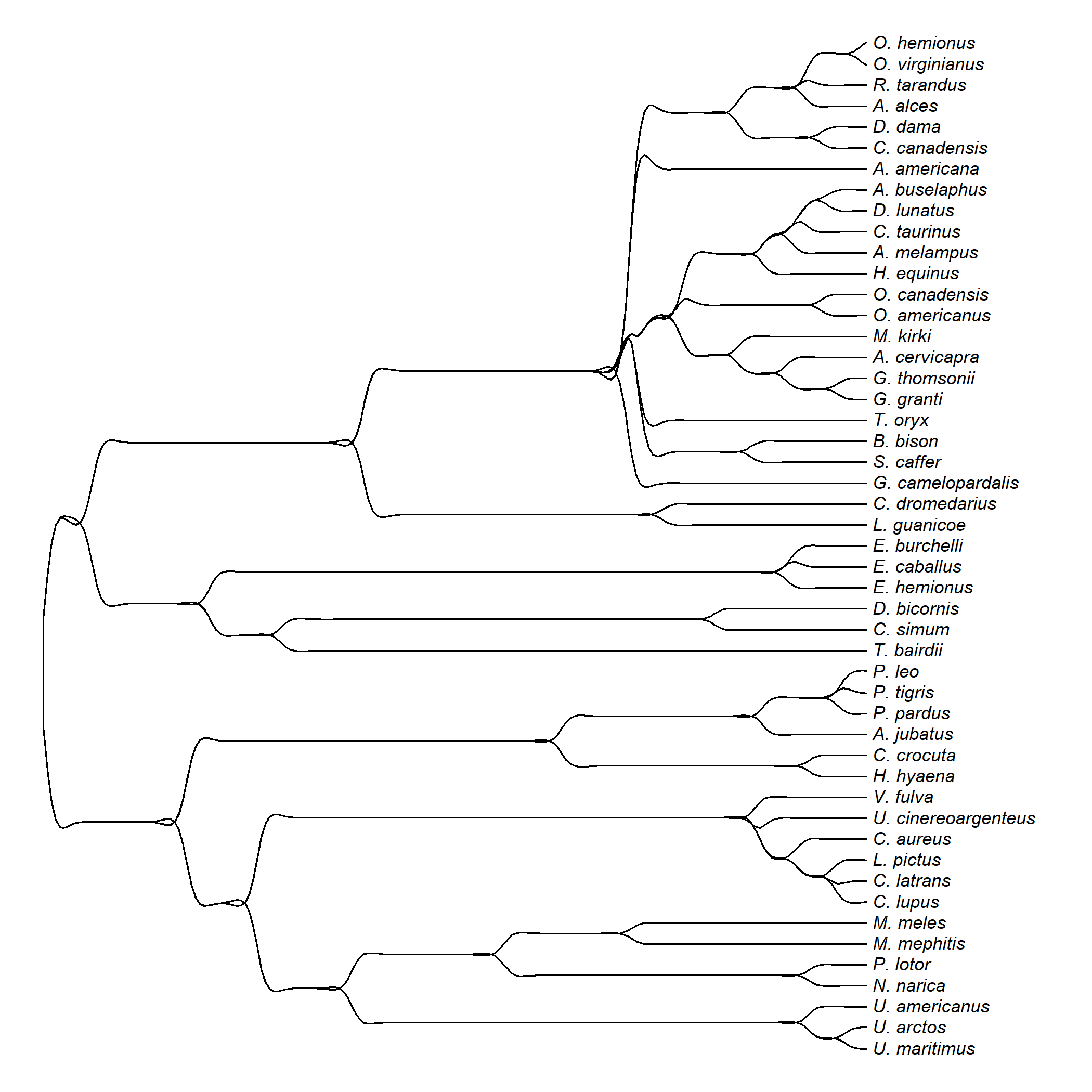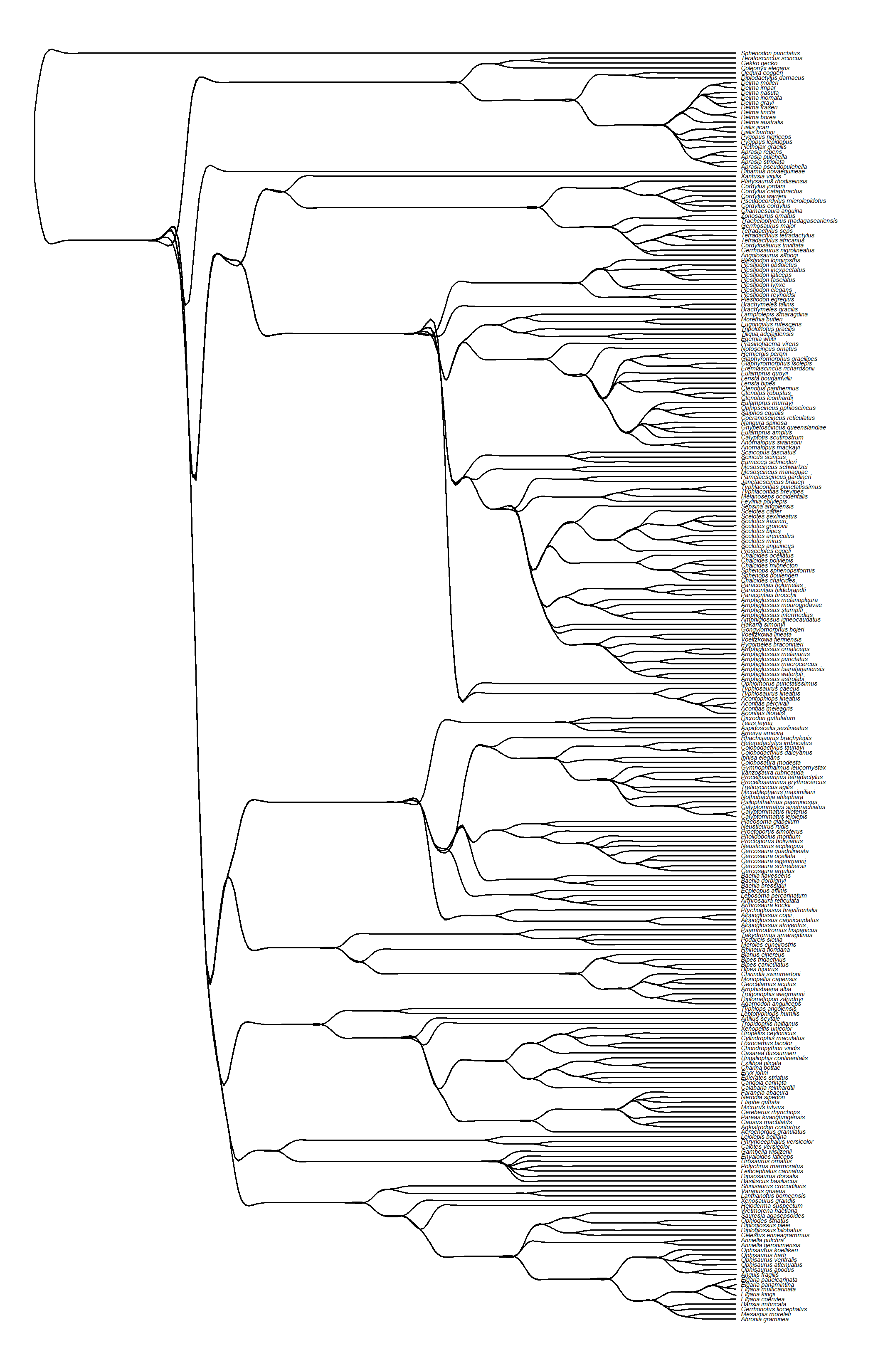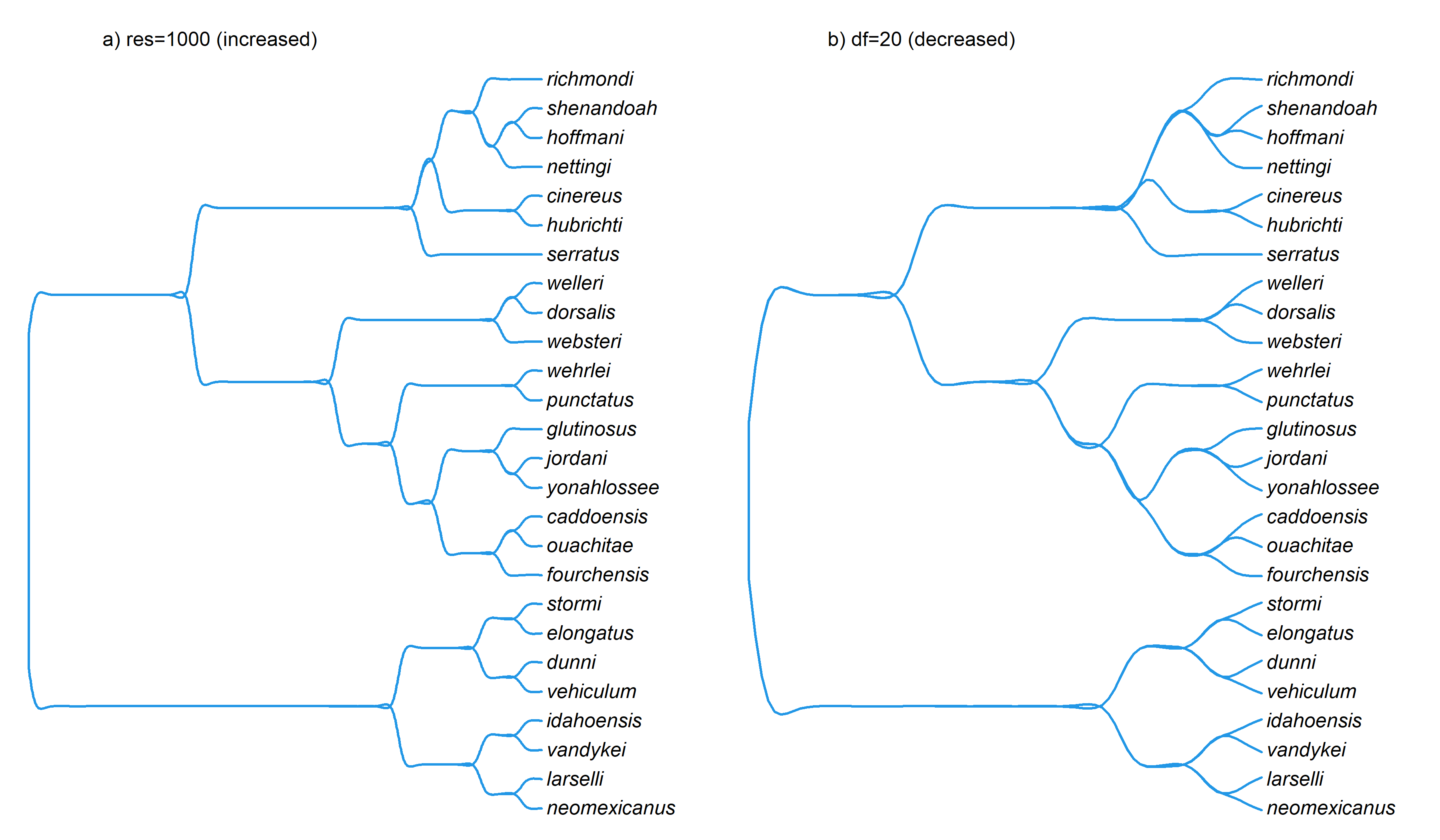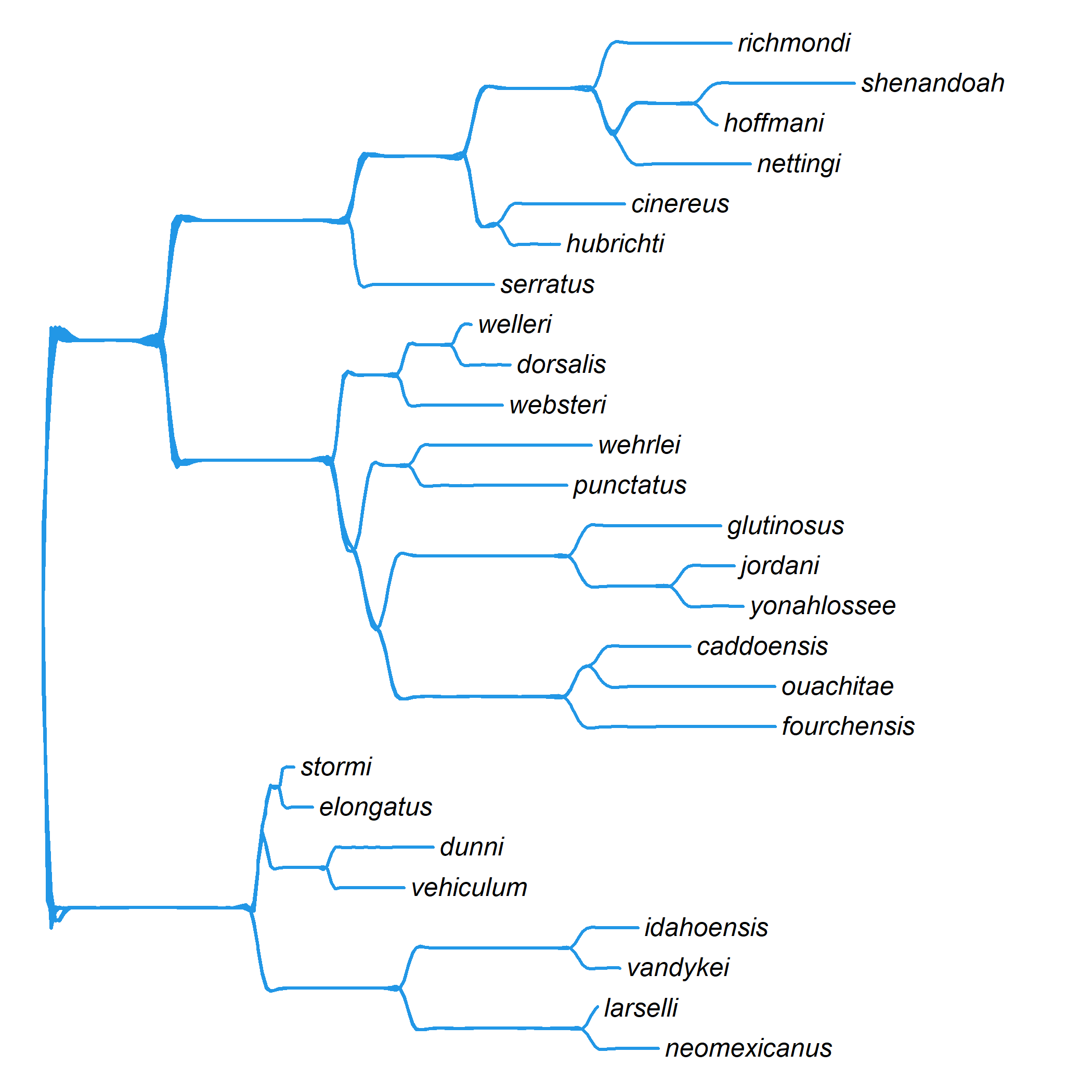## Sunday, January 29, 2023

### Smooth spline phylogram method now in phytools

Recently on my blog I described a fun algorithm for tree plotting that uses cubic spline interpolation to draw the lines through nodes of the phylogeny.

Just for fun, I decided to add this function to phytools. The code can be seen here.

Note that users wanting to update phytools from GitHub will first need to also update ape from it’s GitHub page. This can be done as follows using the remotes CRAN package.

``````remotes::install_github("emmanuelparadis/ape")
remotes::install_github("liamrevell/phytools")
``````

Let’s check to make sure that our phytools version has updated properly. This should show phytools `1.4.0` or above.

``````packageVersion("phytools")
``````
``````##  '1.4.0'
``````

Let’s load phytools and try it out.

``````library(phytools)
data(mammal.tree)
splinePhylogram(mammal.tree,ftype="i",fsize=0.7)
``````That’s pretty need. Let’s try a different, larger tree. This is a phylogeny of 258 squamate reptiles from Brandley et al. (2008 that we also use in our book.

``````squamate.tree<-read.nexus(file="http://www.phytools.org/Rbook/6/squamate.tre")
splinePhylogram(squamate.tree,ftype="i",fsize=0.3)
``````Neat.

Let’s try a smaller tree – but this time I’ll first strip off the edge lengths.

At the same time, I’m also going to play with two optional arguments `res` and `df`. `res` controls the number of points along edges of the tree that are provided to `smooth.spline` for interpolation. `df` is the trace of the smoother matrix: for higher values, a rougher spline will be drawn to get closer to all of the points.

``````data(salamanders)
salamanders\$edge.length<-NULL
par(mfrow=c(1,2))
splinePhylogram(salamanders,ftype="i",color=palette(),res=1000,
lwd=2,mar=c(0.1,0.1,2.1,0.1))
splinePhylogram(salamanders,ftype="i",color=palette(),df=20,
lwd=2,mar=c(0.1,0.1,2.1,0.1))
``````Users can play with these arguments to see what adjusting both at once will do!

Lastly, here’s the same tree – but with random edge lengths, just so we can see how a non-ultrametric tree looks.

``````salamanders\$edge.length<-runif(n=nrow(salamanders\$edge))
splinePhylogram(salamanders,ftype="i",color=palette(),
lwd=2)
``````Kinda weird.